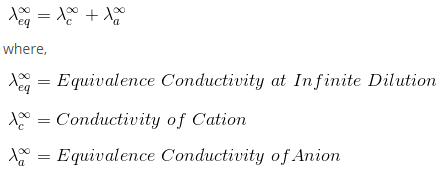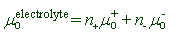# Kohlrausch's Law

Kohlrausch’s law states that the equivalent conductivity of an electrolyte at infinite dilution is equal to the sum of the conductances of the anions and cations. If a salt is dissolved in water, the conductivity of the solution is the sum of the conductances of the anions and cations.The limiting molar conductivity of an electrolyte is the sum of individual contributions of limiting molar conductivities of its constituent ions.Where μ+ and μ- are the limiting molar conductivities of cation and anion respectively.

And n+ and n- are the stoichiometric numbers of positive and negative ions formed during the dissociation of electrolyte.